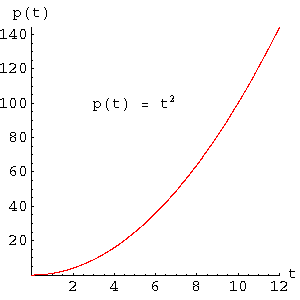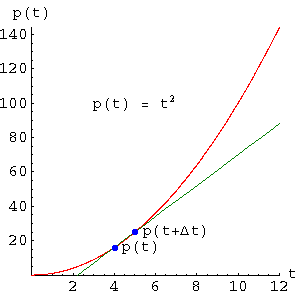Home | Mathematics | * Calculus | * Old Calculus Primer | * Old Calculus Primer 02. A Moving Experience 03. Derivation 04. Integration 05. Volume 1 06. Volume 2 07. Differential Equations 08. Terminal Velocity 09. Nature's Math 10. ToolsShare This Page
Calculus Primer 3: Derivation

P. Lutus Message Page

(double-click any word to see its definition)

Now that we have presented an overview, we can look more closely at some of the elements of Calculus. This page discusses derivation, the operation meant to find a rate of change.

In the previous page we showed a table of values for position and velocity. What this meant was the data points lay on integer seconds, something not usually seen in nature. In real life, data are rarely so coöperative, and neither are mathematical functions. I think this may be an appropriate moment to discuss functions.

Functions
A function is a named abstraction that refers to an arbitrary mathematical operation. A function accepts one or more arguments and returns a result. Here is an example:

(1)    p(t) = t2

We have defined a function p(t) and we have assigned it the operation "t2". But, just as with a letter variable in algebra, this assignment is arbitrary, and the function name may appear in a context for which any function definition is acceptable. In the same way that letters symbolize numbers in algebra, functions symbolize operations in Calculus and other fields of mathematics. In both cases, the substitution of a general symbol for a specific value greatly increases the power and flexibility of the expression in which you find it.

Finding a rate of changeGraph 1: The functionGraph 2: An average rate of changeGraph 3: A specific rate of change
In this example, instead of examining a table of data, let's explore the properties of the function described above. A graph of this function appears as Graph 1.

Let us say the function p(t) describes the position of the car from the previous example with respect to time. Our job is to find out how its position changes as time passes, and use that information to derive its velocity and acceleration.

In the previous section we worked with a table of numbers. We could pick any two numbers and obtain their difference, thus easily deriving a velocity. What happens if we try that same method using a function instead of numbers? Let's apply two time arguments to our function and see what happens. You may remember from the previous page that a rate of change with respect to time is equal to:

 (2) p2 - p1 = vt2 - t1

But in this problem we are using a function, not a variable, so we might write our equation like this:

 (3) p(t2) - p(t1) = vt2 - t1

We need one more refinement to our equation. We are no longer using times that represent indices into a table of values, and we could simplify this equation if we used a single variable to represent the chosen difference in time. Like this:

 (4) p(t+Δt) - p(t) = vΔt

In this new equation, Δt, spoken as "delta t", represents a small increment in time.

Let's start by taking a large time interval, as we did in the previous section using a table of numbers, and see if this will produce something useful. We set Δt = 12, t = 0, and apply equation (4) above:

 (5) p(12) - p(0) = 1212

This result, shown in Graph 2 as a green line with two measured endpoints in blue, creates a single velocity result of 12t (12 times t). But it is clear that there are many other velocities present during the time interval, which we can see by examining the red position curve.

Let's experiment with setting a smaller Δt value and see what happens. An example of this is shown as Graph 3 on this page.

We know from consulting the table from the previous page that the correct velocity value for t = 4 is 8 meters per second. Let's see if we can obtain an approximation for this value by applying equation (4) above using different time increments. Let's set t = 4 and Δt = .1:

 (6) p(4+.1) - p(4) = 8.1.1

Okay, that looks promising. Let's try a smaller value for Δt:

 (7) p(4+.001) - p(4) = 8.001.001

A pattern is emerging. It seems the smaller we make Δt, the more accurate our result becomes, but because Δt is not zero, our result never exactly equals the correct value. How can we make the result agree with the true velocity value of 8?

Let's consider our options. Why not set Δt = 0? Wouldn't that solve the problem? As it turns out, we cannot use a value of zero because that would require us to divide by zero, an operation that is undefined in mathematics. This introduces an important topic in Calculus, the idea of a limit.

The Limit
Although we cannot solve our problem by setting Δt = 0, we can make a statement in which we approach zero. Using words we would say "The limit of a function f(x), as x approaches zero, is equal to y", or using symbols:

 (8) limΔx -> 0 f(x) = y

The limit allows us to make statements about functions we would not otherwise be allowed to make. In this case we cannot say "if Δt equals zero, then our velocity result is equal to 8". But we can say "As Δt approaches zero, our result approaches 8 (or 'is equal to 8 in the limit')."

Deriving a new function
So far, we have used the method of derivatives and the notion of a limit to obtain a numerical result that represents a specific outcome for a specific argument. But a version of the method presented above can be used to obtain a new function that represents the derivative of our original function. The advantages of this should be obvious — we can obtain a function, then use that function to obtain any desired numerical result, for any argument value. This approach is much more useful than acquiring specific numerical results as we did above.

Because this is an introductory Calculus tutorial, we will not dwell very long on this relatively technical topic, and those who don't care to follow an algebraic derivation may wish to skip the next section. But it is important to understand that producing a function as a derivative is much more useful than producing a numerical result.

Let's review what we know. Based on the numerical result we acquired above (using the idea of a limit, at t = 4 our result was approximately 8), we might guess that the derivative of t2 with respect to t is 2t, but if we only examine numerical results, this can never be more than a guess. How do we prove this guess, and how can we create a systematic approach to obtaining a function as a derivative?

We begin with the position function we have been discussing above, p(t) = t2. Our goal is to obtain a new function that is the derivative of this one, a function that provides velocities. We will use the notation p'(t) (note the apostrophe) to stand for the derivative we seek. This same notation can be extended to higher derivatives, for example p''(t) represents the second derivative of p(t), or acceleration.

Let's begin by asserting something we will then prove:

 (9) limΔt -> 0 p(t+Δt) - p(t) = p'(t)Δt

Using words to describe equation (9), we might say "as the limit of Δt approaches 0, the expression at the right approaches the derivative of p(t)", indicated above as p'(t). Note that the assertion above applies to any function, and our use of functional notation allows us to make a general statement about all functions, not just one.

We will now perform a step-by-step process of algebraic manipulation to obtain the derivative of p(t) = t2. As a first step, we temporarily abandon the functional notation, so that this:

 (10) p(t+Δt) - p(t)Δt

Becomes this:

 (11) (t + Δt)2 - t2Δt

For our next step, using high school algebra, we can show that (t + Δt)2 is equivalent to t2 + 2tΔt + Δt2, so we now have:

 (12) t2 + 2tΔt + Δt2 - t2Δt

Simplifying the numerator (where both t2 and -t2 are present), we get:

 (13) 2tΔt + Δt2Δt

Now dividing through by Δt we get:

(14)    2t + Δt

Taking the limit of this expression, we have:

 (15) limΔt -> 0 2t + Δt = 2t

And, as they say, Q.E.D.. As it turns out, derivatives for most functions can be obtained using methods like this.

 Home | Mathematics | * Calculus | * Old Calculus Primer | * Old Calculus Primer 02. A Moving Experience 03. Derivation 04. Integration 05. Volume 1 06. Volume 2 07. Differential Equations 08. Terminal Velocity 09. Nature's Math 10. ToolsShare This Page